Main Content

# tfest

Estimate transfer function

## Syntax

``sys = tfest(data,np)``
``sys = tfest(data,np,nz)``
``sys = tfest(data,np,nz,iodelay)``
``sys = tfest(___,Name,Value)``
``sys = tfest(data,init_sys)``
``sys = tfest(___,opt)``
``[sys,ic] = tfest(___)``

## Description

### Estimate a Transfer Function Model

example

````sys = tfest(data,np)` estimates a continuous-time transfer function `sys` using the time-domain or frequency-domain data `data` and containing `np` poles. The number of zeros in `sys` is `max(np-1,0)`.```

example

````sys = tfest(data,np,nz)` estimates a transfer function containing `nz` zeros.```

example

````sys = tfest(data,np,nz,iodelay)` estimates a transfer function with transport delay for the input-output pairs in `iodelay`.```

example

````sys = tfest(___,Name,Value)` uses additional options specified by one or more name-value pair arguments. You can use this syntax with any of the previous input-argument combinations.```

### Configure Initial Parameters

example

````sys = tfest(data,init_sys)` uses the linear system `init_sys` to configure the initial parameterization of `sys`.```

### Specify Additional Options

example

````sys = tfest(___,opt)` specifies the estimation behavior using the option set `opt`. You can use this syntax with any of the previous input-argument combinations.```

### Return Estimated Initial Conditions

example

````[sys,ic] = tfest(___)` returns the estimated initial conditions as an `initialCondition` object. Use this syntax if you plan to simulate or predict the model response using the same estimation input data and then compare the response with the same estimation output data. Incorporating the initial conditions yields a better match during the first part of the simulation.```

## Examples

collapse all

Load the time-domain system-response data `z1`.

`load iddata1 z1;`

Set the number of poles `np` to `2` and estimate a transfer function.

```np = 2; sys = tfest(z1,np);```

`sys` is an `idtf` model containing the estimated two-pole transfer function.

View the numerator and denominator coefficients of the resulting estimated model `sys`.

`sys.Numerator`
```ans = 1×2 2.4554 176.9856 ```
`sys.Denominator`
```ans = 1×3 1.0000 3.1625 23.1631 ```

To view the uncertainty in the estimates of the numerator and denominator and other information, use `tfdata`.

Load time-domain system response data `z2` and use it to estimate a transfer function that contains two poles and one zero.

```load iddata2 z2; np = 2; nz = 1; sys = tfest(z2,np,nz);```

`sys` is an `idtf` model containing the estimated transfer function.

Load the data `z2`, which is an `iddata` object that contains time-domain system response data.

`load iddata2 z2;`

Estimate a transfer function model `sys` that contains two poles and one zero, and which includes a known transport delay `iodelay`.

```np = 2; nz = 1; iodelay = 0.2; sys = tfest(z2,np,nz,iodelay);```

`sys` is an `idtf` model containing the estimated transfer function, with the `IODelay` property set to 0.2 seconds.

Load time-domain system response data `z2` and use it to estimate a two-pole one-zero transfer function for the system. Specify an unknown transport delay for the transfer function by setting the value of `iodelay` to `NaN`.

```load iddata2 z2; np = 2; nz = 1; iodelay = NaN; sys = tfest(z2,np,nz,iodelay);```

`sys` is an `idtf` model containing the estimated transfer function, whose `IODelay` property is estimated using the data.

Load time-domain system response data `z2`.

`load iddata2 z2`

Estimate a discrete-time transfer function with two poles and one zero. Specify the sample time `Ts` as 0.1 seconds and the transport delay `iodelay` as 2 seconds.

```np = 2; nz = 1; iodelay = 2; Ts = 0.1; sysd = tfest(z2,np,nz,iodelay,'Ts',Ts)```
```sysd = From input "u1" to output "y1": 1.8 z^-1 z^(-2) * ---------------------------- 1 - 1.418 z^-1 + 0.6613 z^-2 Sample time: 0.1 seconds Discrete-time identified transfer function. Parameterization: Number of poles: 2 Number of zeros: 1 Number of free coefficients: 3 Use "tfdata", "getpvec", "getcov" for parameters and their uncertainties. Status: Estimated using TFEST on time domain data "z2". Fit to estimation data: 80.26% FPE: 2.095, MSE: 2.063 ```

By default, the model has no feedthrough, and the numerator polynomial of the estimated transfer function has a zero leading coefficient `b0`. To estimate `b0`, specify the `Feedthrough` property during estimation.

Load the estimation data `z5`.

`load iddata5 z5`

First, estimate a discrete-time transfer function model with two poles, one zero, and no feedthrough. Get the sample time from the `Ts` property of `z5`.

```np = 2; nz = 1; sys = tfest(z5,np,nz,'Ts',z5.Ts);```

The estimated transfer function has the following form:

`$H\left({z}^{-1}\right)=\frac{b1{z}^{-1}+b2{z}^{-2}}{1+a1{z}^{-1}+a2{z}^{-2}}$`

By default, the model has no feedthrough, and the numerator polynomial of the estimated transfer function has a zero leading coefficient `b0`. To estimate `b0`, specify the `Feedthrough` property during estimation.

`sys = tfest(z5,np,nz,'Ts',z5.Ts,'Feedthrough',true);`

The numerator polynomial of the estimated transfer function now has a nonzero leading coefficient:

`$H\left({z}^{-1}\right)=\frac{b0+b1{z}^{-1}+b2{z}^{-2}}{1+a1{z}^{-1}+a2{z}^{-2}}$`

Compare two discrete-time models with and without feedthrough and transport delay.

If there is a delay from the measured input to output, it can be attributed either to a lack of feedthrough or to an actual transport delay. For discrete-time models, absence of feedthrough corresponds to a lag of one sample between the input and output. Estimating a model using `Feedthrough = false` and `iodelay = 0` thus produces a discrete-time system that is equivalent to a system estimated using `Feedthrough = true` and `iodelay = 1`. Both systems show the same time- and frequency-domain responses, for example, on step and Bode plots. However, you get different results if you reduce these models using `balred` or convert them to their continuous-time representations. Therefore, a best practice is to check if the observed delay can be attributed to a transport delay or to a lack of feedthrough.

Estimate a discrete-time model with no feedthrough.

```load iddata1 z1 np = 2; nz = 2; sys1 = tfest(z1,np,nz,'Ts',z1.Ts);```

Because `sys1` has no feedthrough and therefore has a numerator polynomial that beings with ${z}^{-1}$, `sys1` has a lag of one sample. The `IODelay` property is 0.

Estimate another discrete-time model with feedthrough and with a reduction from two zeros to one, incurring a one-sample input-output delay.

`sys2 = tfest(z1,np,nz-1,1,'Ts',z1.Ts,'Feedthrough',true);`

Compare the Bode responses of the models.

`bode(sys1,sys2);`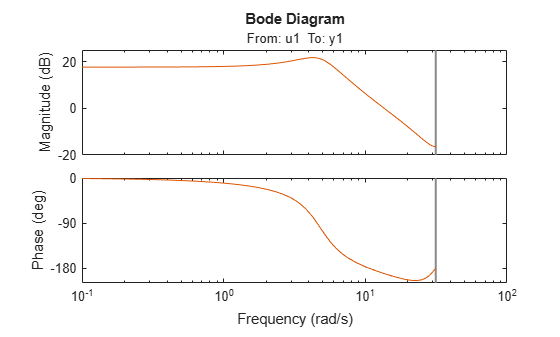The discrete equations that underlie sys`1` and sys`2` are equivalent, and so are the Bode responses.

Convert the models to continuous time and compare the Bode responses for these models.

```sys1c = d2c(sys1); sys2c = d2c(sys2); bode(sys1c,sys2c); legend```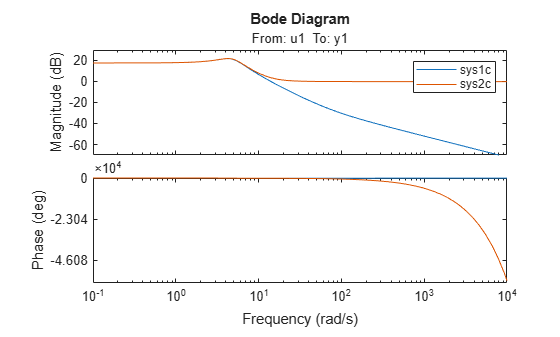As the plot shows, the Bode responses of the two models do not match when you convert them to continuous time. When there is no feedthrough, as with `sys1c`, there must be some lag. When there is feedthrough, as with `sys2c`, there can be no lag. Continuous-time feedthrough maps to discrete-time feedthrough. Continuous-time lag maps to discrete-time delays.

Estimate a two-input, one-output discrete-time transfer function with a delay of two 2 samples on the first input and zero samples on the second input. Both inputs have no feedthrough.

Load the data and split the data into estimation and validation data sets.

```load iddata7 z7 ze = z7(1:300); zv = z7(200:400);```

Estimate a two-input, one-output transfer function with two poles and one zero for each input-to-output transfer function.

```Lag = [2;0]; Ft = [false,false]; model = tfest(ze,2,1,'Ts',z7.Ts,'Feedthrough',Ft,'InputDelay',Lag);```

The `Feedthrough` value you choose dictates whether the leading numerator coefficient is zero (no feedthrough) or not (nonzero feedthrough). Delays are generally expressed separately using the `InputDelay` or `IODelay` property. This example uses `InputDelay` only to express the delays.

Validate the estimated model. Exclude the data outliers for validation.

```I = 1:201; I(114:118) = []; opt = compareOptions('Samples',I); compare(zv,model,opt)```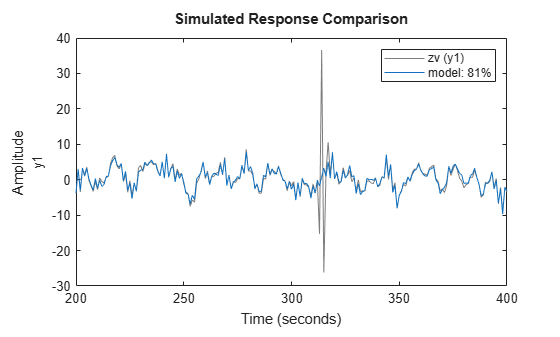Identify a 15th order transfer function model by using regularized impulse response estimation.

Load the data.

`load regularizationExampleData m0simdata;`

Obtain a regularized impulse response (FIR) model.

```opt = impulseestOptions('RegularizationKernel','DC'); m0 = impulseest(m0simdata,70,opt);```

Convert the model into a transfer function model after reducing the order to 15.

`m = idtf(balred(idss(m0),15));`

Compare the model output with the data.

`compare(m0simdata,m);`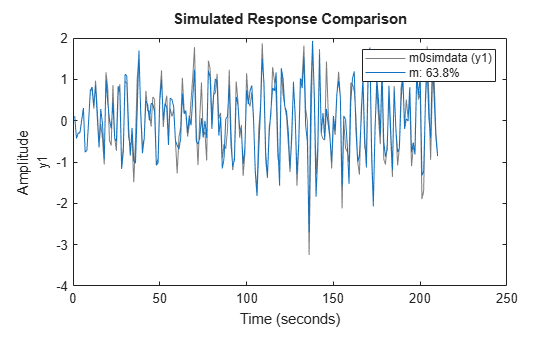Create an option set for `tfest` that specifies the initialization and search methods. Also set the display option, which specifies that the loss-function values for each iteration be shown.

`opt = tfestOptions('InitializeMethod','n4sid','Display','on','SearchMethod','lsqnonlin');`

Load time-domain system response data `z2` and use it to estimate a transfer function with two poles and one zero. Specify `opt` for the estimation options.

```load iddata2 z2; np = 2; nz = 1; iodelay = 0.2; sys = tfest(z2,np,nz,iodelay,opt);```

`sys` is an `idtf` model containing the estimated transfer function.

Load the time-domain system response data `z2`, and use it to estimate a two-pole, one-zero transfer function. Specify an input delay.

```load iddata2 z2; np = 2; nz = 1; input_delay = 0.2; sys = tfest(z2,np,nz,'InputDelay',input_delay);```

`sys` is an `idtf` model containing the estimated transfer function with an input delay of 0.2 seconds.

Use `bode` to obtain the magnitude and phase response for the following system:

`$H\left(s\right)=\frac{s+0.2}{{s}^{3}+2{s}^{2}+s+1}$`

Use 100 frequency points, ranging from 0.1 rad/s to 10 rad/s, to obtain the frequency-response data. Use `frd` to create a frequency-response data object.

```freq = logspace(-1,1,100); [mag,phase] = bode(tf([1 0.2],[1 2 1 1]),freq); data = frd(mag.*exp(1j*phase*pi/180),freq);```

Estimate a three-pole, one-zero transfer function using `data`.

```np = 3; nz = 1; sys = tfest(data,np,nz);```

`sys` is an `idtf` model containing the estimated transfer function.

Load the time-domain system response data `co2data`, which contains the data from two experiments, each with two inputs and one output. Convert the data from the first experiment into an `iddata` object `data` with a sample time of 0.5 seconds.

```load co2data; Ts = 0.5; data = iddata(Output_exp1,Input_exp1,Ts);```

Specify estimation options for the search method and the input and output offsets. Also specify the maximum number of search iterations.

```opt = tfestOptions('SearchMethod','gna'); opt.InputOffset = [170;50]; opt.OutputOffset = mean(data.y(1:75)); opt.SearchOptions.MaxIterations = 50;```

Estimate a transfer function using the measured data and the estimation option set `opt`. Specify the transport delays from the inputs to the output.

```np = 3; nz = 1; iodelay = [2 5]; sys = tfest(data,np,nz,iodelay,opt);```

`iodelay` specifies the input-to-output delay from the first and second inputs to the output as 2 seconds and 5 seconds, respectively.

`sys` is an `idtf` model containing the estimated transfer function.

Load time-domain system response data and use it to estimate a transfer function for the system. Specify the known and unknown transport delays.

```load co2data; Ts = 0.5; data = iddata(Output_exp1,Input_exp1,Ts);```

`data` is an `iddata` object with two input channels and one output channels, and which has a sample rate of 0.5 seconds.

Create an option set `opt`. Specify estimation options for the search method and the input and output offsets. Also specify the maximum number of search iterations.

```opt = tfestOptions('Display','on','SearchMethod','gna'); opt.InputOffset = [170; 50]; opt.OutputOffset = mean(data.y(1:75)); opt.SearchOptions.MaxIterations = 50;```

Specify the unknown and known transport delays in `iodelay`, using `2` for a known delay of 2 seconds and `nan` for the unknown delay. Estimate the transfer function using `iodelay` and `opt`.

```np = 3; nz = 1; iodelay = [2 nan]; sys = tfest(data,np,nz,iodelay,opt);```

`sys` is an `idtf` model containing the estimated transfer function.

Create a transfer function model with the expected numerator and denominator structure and delay constraints.

In this example, the experiment data consists of two inputs and one output. Both transport delays are unknown and have an identical upper bound. Additionally, the transfer functions from both inputs to the output are identical in structure.

```init_sys = idtf(NaN(1,2),[1,NaN(1,3)],'IODelay',NaN); init_sys.Structure(1).IODelay.Free = true; init_sys.Structure(1).IODelay.Maximum = 7;```

`init_sys` is an `idtf` model describing the structure of the transfer function from one input to the output. The transfer function consists of one zero, three poles, and a transport delay. The use of `NaN` indicates unknown coefficients.

`init_sys.Structure(1).IODelay.Free = true` indicates that the transport delay is not fixed.

`init_sys.Structure(1).IODelay.Maximum = 7` sets the upper bound for the transport delay to 7 seconds.

Specify the transfer function from both inputs to the output.

`init_sys = [init_sys,init_sys];`

Load time-domain system response data and use it to estimate a transfer function. Specify options in the `tfestOptions` option set `opt`.

```load co2data; Ts = 0.5; data = iddata(Output_exp1,Input_exp1,Ts); opt = tfestOptions('Display','on','SearchMethod','gna'); opt.InputOffset = [170;50]; opt.OutputOffset = mean(data.y(1:75)); opt.SearchOptions.MaxIterations = 50; sys = tfest(data,init_sys,opt);```

`sys` is an `idtf` model containing the estimated transfer function.

Analyze the estimation result by comparison. Create a `compareOptions` option set `opt2` and specify input and output offsets, and then use `compare`.

```opt2 = compareOptions; opt2.InputOffset = opt.InputOffset; opt2.OutputOffset = opt.OutputOffset; compare(data,sys,opt2)```Estimate a multiple-input, single-output transfer function containing different numbers of poles for input-output pairs for given data.

Obtain frequency-response data.

For example, use `frd` to create a frequency-response data model for the following system:

`$G=\left[\begin{array}{c}{e}^{-4s}\frac{s+2}{{s}^{3}+2{s}^{2}+4s+5}\\ {e}^{-0.6s}\frac{5}{{s}^{4}+2{s}^{3}+{s}^{2}+s}\end{array}\right]$`

Use 100 frequency points, ranging from 0.01 rad/s to 100 rad/s, to obtain the frequency-response data.

```G = tf({[1 2],},{[1 2 4 5],[1 2 1 1 0]},0,'IODelay',[4 0.6]); data = frd(G,logspace(-2,2,100));```

`data` is an `frd` object containing the continuous-time frequency response for `G`.

Estimate a transfer function for `data`.

``` np = [3 4]; nz = [1 0]; iodelay = [4 0.6]; sys = tfest(data,np,nz,iodelay);```

`np` specifies the number of poles in the estimated transfer function. The first element of `np` indicates that the transfer function from the first input to the output contains three poles. Similarly, the second element of `np` indicates that the transfer function from the second input to the output contains four poles.

`nz` specifies the number of zeros in the estimated transfer function. The first element of `nz` indicates that the transfer function from the first input to the output contains one zero. Similarly, the second element of `np` indicates that the transfer function from the second input to the output does not contain any zeros.

`iodelay` specifies the transport delay from the first input to the output as 4 seconds. The transport delay from the second input to the output is specified as 0.6 seconds.

`sys` is an `idtf` model containing the estimated transfer function.

Estimate a transfer function describing an unstable system using frequency-response data.

Use `idtf` to construct a transfer function model `G` of the following system:

`$G=\left[\begin{array}{c}\frac{s+2}{{s}^{3}+2{s}^{2}+4s+5}\\ \frac{5}{{s}^{4}+2{s}^{3}+{s}^{2}+s+1}\end{array}\right]$`

`G = idtf({[1 2], 5},{[1 2 4 5],[1 2 1 1 1]});`

Use `idfrd` to obtain a frequency-response data model `data` for `G`. Specify 100 frequency points ranging from 0.01 rad/s to 100 rad/s.

`data = idfrd(G,logspace(-2,2,100));`

`data` is an `idfrd` object.

Estimate a transfer function for `data`.

```np = [3 4]; nz = [1 0]; sys = tfest(data,np,nz);```

`np` specifies the number of poles in the estimated transfer function. The first element of `np` indicates that the transfer function from the first input to the output contains three poles. Similarly, the second element of `np` indicates that the transfer function from the second input to the output contains four poles.

`nz` specifies the number of zeros in the estimated transfer function. The first element of `nz` indicates that the transfer function from the first input to the output contains one zero. Similarly, the second element of `nz` indicates that the transfer function from the second input to the output does not contain any zeros.

`sys` is an `idtf` model containing the estimated transfer function.

`pole(sys)`
```ans = 7×1 complex -1.5260 + 0.0000i -0.2370 + 1.7946i -0.2370 - 1.7946i -1.4656 + 0.0000i -1.0000 + 0.0000i 0.2328 + 0.7926i 0.2328 - 0.7926i ```

`sys` is an unstable system, as the pole display indicates.

Load the high-density frequency-response measurement data. The data corresponds to an unstable process maintained at equilibrium using feedback control.

`load HighModalDensityData FRF f`

Package the data as an `idfrd` object for identification and find the Bode magnitude response.

```G = idfrd(permute(FRF,[2 3 1]),f,0,'FrequencyUnit','Hz'); bodemag(G)```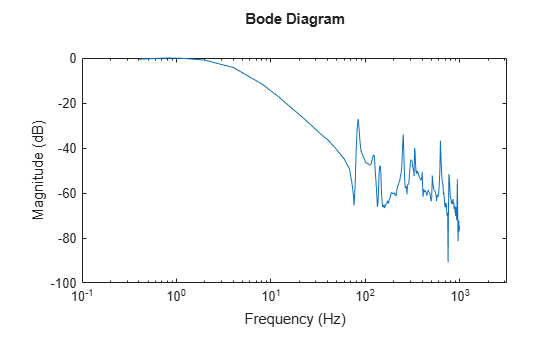Estimate a transfer function with 32 poles and 32 zeros, and compare the Bode magnitude response.

```sys = tfest(G,32,32); bodemag(G, sys) xlim([0.01,2e3]) legend```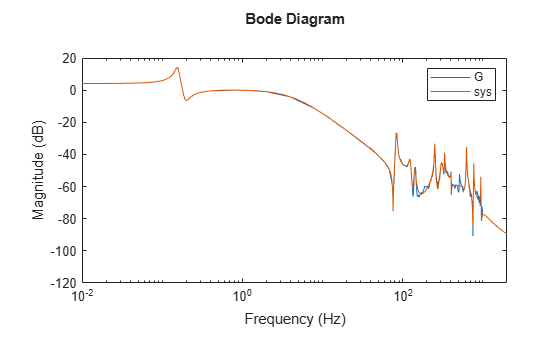Load and plot the data.

```load iddata1ic z1i plot(z1i)```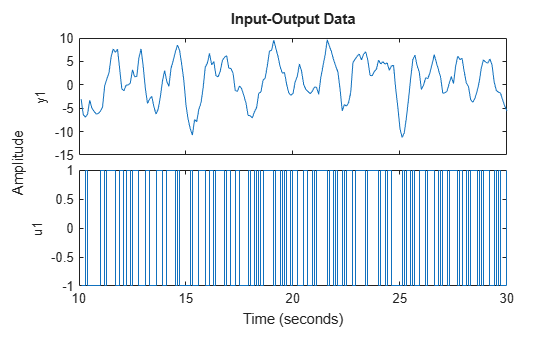Examine the initial value of the output data `y(1)`.

`ystart = z1i.y(1)`
```ystart = -3.0491 ```

The measured output does not start at 0.

Estimate a second-order transfer function `sys` and return the estimated initial condition `ic`.

```[sys,ic] = tfest(z1i,2,1); ic```
```ic = initialCondition with properties: A: [2x2 double] X0: [2x1 double] C: [0.2957 5.2441] Ts: 0 ```

`ic` is an `initialCondition` object that encapsulates the free response of `sys`, in state-space form, to the initial state vector in `X0`.

Simulate `sys` using the estimation data but without incorporating the initial conditions. Plot the simulated output with the measured output.

```y_no_ic = sim(sys,z1i); plot(y_no_ic,z1i) legend('Model Response','Output Data')```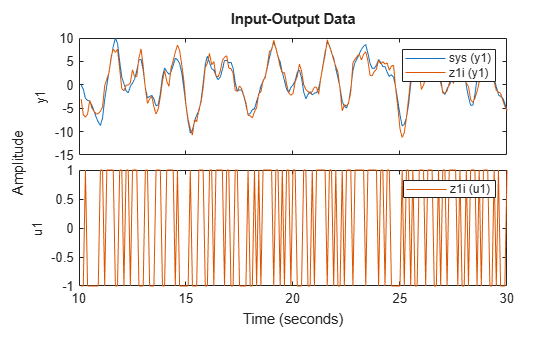The measured and simulated outputs do not agree at the beginning of the simulation.

Incorporate the initial condition into the `simOptions` option set.

```opt = simOptions('InitialCondition',ic); y_ic = sim(sys,z1i,opt); plot(y_ic,z1i) legend('Model Response','Output Data')```The simulation combines the model response to the input signal with the free response to the initial condition. The measured and simulated outputs now have better agreement at the beginning of the simulation. This initial condition is valid only for the estimation data `z1i`.

## Input Arguments

collapse all

Estimation data, specified as an `iddata` object, an `frd` object, or an `idfrd` object.

For time-domain estimation, `data` must be an `iddata` object containing the input and output signal values.

Time-series models, which are models that contain no measured inputs, cannot be estimated using `tfest`. Use `ar`, `arx`, or `armax` for time-series models instead.

For frequency-domain estimation, `data` can be one of the following:

• Recorded frequency response data (`frd` (Control System Toolbox) or `idfrd`)

• `iddata` object with properties specified as follows:

• `InputData` — Fourier transform of the input signal

• `OutputData` — Fourier transform of the output signal

• `Domain``'Frequency'`

Estimation data must be uniformly sampled.

For multiexperiment data, the sample times and intersample behavior of all the experiments must match.

You can estimate both continuous-time and discrete-time models (of sample time matching that of `data`) using time-domain data and discrete-time frequency-domain data. You can estimate only continuous-time models using continuous-time frequency-domain data.

Number of poles in the estimated transfer function, specified as a nonnegative integer or a matrix.

For systems that have multiple inputs and/or multiple outputs, you can apply either a global value or individual values of `np` to the input-output pairs, as follows:

• Same number of poles for every pair — Specify `np` as a scalar.

• Individual number of poles for each pair — Specify `np` as an ny-by-nu matrix. ny is the number of outputs and nu is the number of inputs.

For an example, see Estimate Transfer Function Model by Specifying Number of Poles.

Number of zeros in the estimated transfer function, specified as a nonnegative integer or a matrix.

For systems that have multiple inputs, multiple outputs, or both, you can apply either a global value or individual values of `nz` to the input-output pairs, as follows:

• Same number of poles for every pair — Specify `nz` as a scalar.

• Individual number of poles for each pair — Specify `nz` as an ny-by-nu matrix. ny is the number of outputs and nu is the number of inputs.

For a continuous-time model estimated using discrete-time data, set `nz` <= `np`.

For discrete-time model estimation, specify `nz` as the number of zeros of the numerator polynomial of the transfer function. For example, `tfest(data,2,1,'Ts',data.Ts)` estimates a transfer function of the form ${b}_{1}{z}^{-1}/\left(1+{a}_{1}{z}^{-1}+{b}_{2}{z}^{-2}\right)$, while `tfest(data,2,2,'Ts',data.Ts)` estimates $\left({b}_{1}{z}^{-1}+{b}_{2}{z}^{-2}\right)/\left(1+{a}_{1}{z}^{-1}+{b}_{2}{z}^{-2}\right)$. Here, z-1 is the Z-transform lag variable. For more information about discrete-time transfer functions, see Discrete-Time Representation. For an example, see Estimate Discrete-Time Transfer Function.

Transport delay, specified as a nonnegative integer, an `NaN` scalar, or a matrix.

For continuous-time systems, specify transport delays in the time unit stored in the `TimeUnit` property of `data`. For discrete-time systems, specify transport delays as integers denoting delays of a multiple of the sample time `Ts`.

For a MIMO system with Ny outputs and Nu inputs, set `iodelay` to an Ny-by-Nu array. Each entry of this array is a numerical value that represents the transport delay for the corresponding input-output pair. You can also set `iodelay` to a scalar value to apply the same delay to all input-output pairs.

The specified values are treated as fixed delays. To denote unknown transport delays, specify `NaN` in the `iodelay` matrix.

Use `[]` or `0` to indicate that there is no transport delay.

For an example, see Estimate Transfer Function Containing Known Transport Delay.

Estimation options, specified as an `tfestOptions` option set. Options specified by `opt` include:

• Estimation objective

• Handling of initial conditions

• Numerical search method to be used in estimation

For an example, see Estimate Transfer Function Using Estimation Option Set.

Linear system that configures the initial parameterization of `sys`, specified as an `idtf` model or as a structure. You obtain `init_sys` either by performing an estimation using measured data or by direct construction.

If `init_sys` is an `idtf` model, `tfest` uses the parameter values of `init_sys` as the initial guess for estimating `sys`.

Use the `Structure` property of `init_sys` to configure initial parameter values and constraints for the numerator, denominator, and transport lag. For instance:

• To specify an initial guess for the A matrix of `init_sys`, set `init_sys.Structure.Numerator.Value` to the initial guess.

• To specify constraints for the B matrix of `init_sys`:

• Set `init_sys.Structure.Numerator.Minimum` to the minimum numerator coefficient values.

• Set `init_sys.Structure.Numerator.Maximum` to the maximum numerator coefficient values.

• Set `init_sys.Structure.Numerator.Free` to indicate which numerator coefficients are free for estimation.

For an example, see Estimate Transfer Function with Unknown, Constrained Transport Delays.

If `init_sys` is not an `idtf` model, the software first converts `init_sys` to a transfer function. `tfest` uses the parameters of the resulting model as the initial guess for estimation.

If you do not specify `opt`, and `init_sys` was obtained by estimation rather than construction, then the software uses estimation options from `init_sys.Report.OptionsUsed`.

### Name-Value Pair Arguments

Specify optional comma-separated pairs of `Name,Value` arguments. `Name` is the argument name and `Value` is the corresponding value. `Name` must appear inside quotes. You can specify several name and value pair arguments in any order as `Name1,Value1,...,NameN,ValueN`.

Example: `sys = tfest(data,np,nz,'Ts',0.1)`

Sample time of the estimated model, specified as the comma-separated pair consisting of `'Ts'` and either `0` or a positive scalar.

• For continuous-time models, specify `'Ts'` as `0`.

• For discrete-time models, specify `'Ts'` as the data sample time in the units stored in the `TimeUnit` property. In the discrete case, `np` and `nz` refer to the number of roots of z-1 for the numerator and denominator polynomials.

For an example, see Estimate Discrete-Time Transfer Function.

Input delay for each input channel, specified as the comma-separated pair consisting of `'InputDelay'` and a numeric vector.

• For continuous-time models, specify `'InputDelay'` in the time units stored in the `TimeUnit` property.

• For discrete-time models, specify `'InputDelay'` in integer multiples of the sample time `Ts`. For example, setting `'InputDelay'` to `3` specifies a delay of three sampling periods.

For a system with Nu inputs, set `InputDelay` to an Nu-by-1 vector. Each entry of this vector is a numerical value that represents the input delay for the corresponding input channel.

To apply the same delay to all channels, specify `InputDelay` as a scalar.

For an example, see Specify Model Properties of Estimated Transfer Function.

Feedthrough for discrete-time transfer functions, specified as the comma-separated pair consisting of `'Feedthrough'` a logical scalar or an Ny-by-Nu logical matrix. Ny is the number of outputs and Nu is the number of inputs. To use the same feedthrough for all input-output channels, specify `Feedthrough` as a scalar.

Consider a discrete-time model with two poles and three zeros:

$H\left({z}^{-1}\right)=\frac{b0+b1{z}^{-1}+b2{z}^{-2}+b3{z}^{-3}}{1+a1{z}^{-1}+a2{z}^{-2}}$

When the model has direct feedthrough, b0 is a free parameter whose value is estimated along with the rest of the model parameters b1, b2, b3, a1, and a2. When the model has no feedthrough, b0 is fixed to zero. For an example, see Estimate Discrete-Time Transfer Function with Feedthrough.

## Output Arguments

collapse all

Identified transfer function, returned as an `idtf` object. This model is created using the specified model orders, delays, and estimation options.

Information about the estimation results and options used is stored in the `Report` property of the model. `Report` has the following fields.

Report FieldDescription
`Status`

Summary of the model status, which indicates whether the model was created by construction or obtained by estimation.

`Method`

Estimation command used.

`InitializeMethod`

Algorithm used to initialize the numerator and denominator for estimation of continuous-time transfer functions using time-domain data, returned as one of the following values:

• `'iv'` — Instrument Variable approach

• `'svf'` — State Variable Filters approach

• `'gpmf'` — Generalized Poisson Moment Functions approach

• `'n4sid'` — Subspace state-space estimation approach

This field is especially useful to view the algorithm used when the `InitializeMethod` option in the estimation option set is `'all'`.

`N4Weight`

Weighting matrices used in the singular-value decomposition step when `InitializeMethod` is `'n4sid'`, returned as one of the following values:

• `'MOESP'` — Use the MOESP algorithm by Verhaegen.

• `'CVA'` — Use the canonical variate algorithm (CVA) by Larimore.

• `'SSARX'` — Use a subspace identification method that uses an ARX estimation-based algorithm to compute the weighting.

This field is especially useful to view the weighting matrices used when the `N4Weight` option in the estimation option set is `'auto'`.

`N4Horizon`

Forward and backward prediction horizons used when `InitializeMethod` is `'n4sid'`, returned as a row vector with three elements — `[r sy su]`, where `r` is the maximum forward prediction horizon. `sy` is the number of past outputs, and `su` is the number of past inputs that are used for the predictions.

`InitialCondition`

Handling of initial conditions during model estimation, returned as one of the following values:

• `'zero'` — The initial conditions were set to zero.

• `'estimate'` — The initial conditions were treated as independent estimation parameters.

• `'backcast'` — The initial conditions were estimated using the best least squares fit.

This field is especially useful to view how the initial conditions were handled when the `InitialCondition` option in the estimation option set is `'auto'`.

`Fit`

Quantitative assessment of the estimation, returned as a structure. See Loss Function and Model Quality Metrics for more information on these quality metrics. The structure has the following fields:

FieldDescription
`FitPercent`

Normalized root mean squared error (NRMSE) measure of how well the response of the model fits the estimation data, expressed as the percentage `fit` = 100(1-NRMSE).

`LossFcn`

Value of the loss function when the estimation completes.

`MSE`

Mean squared error (MSE) measure of how well the response of the model fits the estimation data.

`FPE`

Final prediction error for the model.

`AIC`

Raw Akaike Information Criteria (AIC) measure of model quality.

`AICc`

Small sample-size corrected AIC.

`nAIC`

Normalized AIC.

`BIC`

Bayesian Information Criteria (BIC).

`Parameters`

Estimated values of model parameters.

`OptionsUsed`

Option set used for estimation. If no custom options were configured, this is a set of default options. See `polyestOptions` for more information.

`RandState`

State of the random number stream at the start of estimation. Empty, `[]`, if randomization was not used during estimation. For more information, see `rng`.

`DataUsed`

Attributes of the data used for estimation, returned as a structure with the following fields:

FieldDescription
`Name`

Name of the data set.

`Type`

Data type.

`Length`

Number of data samples.

`Ts`

Sample time.

`InterSample`

Input intersample behavior, returned as one of the following values:

• `'zoh'` — Zero-order hold maintains a piecewise-constant input signal between samples.

• `'foh'` — First-order hold maintains a piecewise-linear input signal between samples.

• `'bl'` — Band-limited behavior specifies that the continuous-time input signal has zero power above the Nyquist frequency.

`InputOffset`

Offset removed from time-domain input data during estimation. For nonlinear models, it is `[]`.

`OutputOffset`

Offset removed from time-domain output data during estimation. For nonlinear models, it is `[]`.

`Termination`

Termination conditions for the iterative search used for prediction error minimization, returned as a structure with the following fields:

FieldDescription
`WhyStop`

Reason for terminating the numerical search.

`Iterations`

Number of search iterations performed by the estimation algorithm.

`FirstOrderOptimality`

$\infty$-norm of the gradient search vector when the search algorithm terminates.

`FcnCount`

Number of times the objective function was called.

`UpdateNorm`

Norm of the gradient search vector in the last iteration. Omitted when the search method is `'lsqnonlin'` or `'fmincon'`.

`LastImprovement`

Criterion improvement in the last iteration, expressed as a percentage. Omitted when the search method is `'lsqnonlin'` or `'fmincon'`.

`Algorithm`

Algorithm used by `'lsqnonlin'` or `'fmincon'` search method. Omitted when other search methods are used.

For estimation methods that do not require numerical search optimization, the `Termination` field is omitted.

For more information on using `Report`, see Estimation Report.

Estimated initial conditions, returned as an `initialCondition` object or an object array of `initialCondition` values.

• For a single-experiment data set, `ic` represents, in state-space form, the free response of the transfer function model (A and C matrices) to the estimated initial states (x0).

• For a multiple-experiment data set with Ne experiments, `ic` is an object array of length Ne that contains one set of `initialCondition` values for each experiment.

If `tfest` returns `ic` values of `0` and the you know that you have non-zero initial conditions, set the `'InitialCondition'` option in `tfestOptions` to `'estimate'` and pass the updated option set to `tfest`. For example:

```opt = tfestOptions('InitialCondition,'estimate') [sys,ic] = tfest(data,np,nz,opt)```
The default `'auto'` setting of `'InitialCondition'` uses the `'zero'` method when the initial conditions have a negligible effect on the overall estimation-error minimization process. Specifying `'estimate'` ensures that the software estimates values for `ic`.

For more information, see `initialCondition`. For an example of using this argument, see Obtain and Apply Estimated Initial Conditions.

## Algorithms

collapse all

The details of the estimation algorithms used by `tfest` vary depending on various factors, including the sampling of the estimated model and the estimation data.

### Continuous-Time Transfer Function Estimation Using Time-Domain Data

Parameter Initialization

The estimation algorithm initializes the estimable parameters using the method specified by the `InitializeMethod` estimation option. The default method is the Instrument Variable (IV) method.

The State-Variable Filters (SVF) approach and the Generalized Poisson Moment Functions (GPMF) approach to continuous-time parameter estimation use prefiltered data  . The constant $\frac{1}{\lambda }$ in  and  corresponds to the initialization option (`InitializeOptions`) field `FilterTimeConstant`. IV is the simplified refined IV method and is called SRIVC in . This method has a prefilter that is the denominator of the current model, initialized with SVF. This prefilter is iterated up to `MaxIterations` times, until the model change is less than `Tolerance`. `MaxIterations` and `Tolerance` are options that you can specify using the `InitializeOptions` structure. The `'n4sid'` initialization option estimates a discrete-time model, using the N4SID estimation algorithm, that it transforms to continuous-time using `d2c`.

Use `tfestOptions` to create the option set used to estimate a transfer function.

Parameter Update

The initialized parameters are updated using a nonlinear least-squares search method, specified by the `SearchMethod` estimation option. The objective of the search method is to minimize the weighted prediction error norm.

### Discrete-Time Transfer Function Estimation Using Time-Domain Data

For discrete-time data, `tfest` uses the same algorithm as `oe` to determine the numerator and denominator polynomial coefficients. In this algorithm, the initialization is performed using `arx`, followed by nonlinear least-squares search-based updates to minimize a weighted prediction error norm.

### Continuous-Time Transfer Function Estimation Using Continuous-Time Frequency-Domain Data

The estimation algorithm performs the following tasks:

1. Perform a bilinear mapping to transform the domain (frequency grid) of the transfer function. For continuous-time models, the imaginary axis is transformed to the unit disk. For discrete-time models, the original domain unit disk is transformed to another unit disk.

2. Perform S-K iterations  to solve a nonlinear least-squares problem — Consider a multi-input single-output system. The nonlinear least-squares problem is to minimize the following loss function:

`$\underset{D,{N}_{i}}{\text{minimize}}\sum _{k=1}^{{n}_{f}}{|W\left({\omega }_{k}\right)\left(y\left({\omega }_{k}\right)-\sum _{i=1}^{{n}_{u}}\frac{{N}_{i}\left({\omega }_{k}\right)}{D\left({\omega }_{k}\right)}{u}_{i}\left({\omega }_{k}\right)\right)|}^{2}$`

Here, W is a frequency-dependent weight that you specify. D is the denominator of the transfer function model that is to be estimated, and Ni is the numerator corresponding to the ith input. y and u are the measured output and input data, respectively. nf and nu are the number of frequencies and inputs, and w is the frequency. Rearranging the terms gives

`$\underset{D,{N}_{i}}{\text{minimize}}\sum _{k=1}^{{n}_{f}}{|\frac{W\left({\omega }_{k}\right)}{D\left({\omega }_{k}\right)}\left(D\left({\omega }_{k}\right)y\left({\omega }_{k}\right)-\sum _{i=1}^{{n}_{u}}{N}_{i}\left({\omega }_{k}\right){u}_{i}\left({\omega }_{k}\right)\right)|}^{2}$`

To perform the S-K iterations, the algorithm iteratively solves

`$\underset{{D}_{m},{N}_{i,m}}{\text{minimize}}\sum _{k=1}^{{n}_{f}}{|\frac{W\left({\omega }_{k}\right)}{{D}_{m-1}\left({\omega }_{k}\right)}\left({D}_{m}\left({\omega }_{k}\right)y\left({\omega }_{k}\right)-\sum _{i=1}^{{n}_{u}}{N}_{i,m}\left({\omega }_{k}\right){u}_{i}\left({\omega }_{k}\right)\right)|}^{2}$`

Here, m is the current iteration, and Dm-1(ω) is the denominator response identified at the previous iteration. Now each step of the iteration is a linear least-squares problem, where the identified parameters capture the responses Dm(ω) and Ni,m(ω) for i = 1,2,...nu. The iteration is initialized by choosing D0(ω) = 1.

• The first iteration of the algorithm identifies D1(ω). The D1(ω) and Ni,1(ω) polynomials are expressed in monomial basis.

• The second and following iterations express the polynomials Dm(ω) and Ni,m(ω) in terms of orthogonal rational basis functions on the unit disk. These basis functions have the form

`${B}_{j,m}\left(\omega \right)=\left(\frac{\sqrt{1-{|{\lambda }_{j,m-1}|}^{2}}}{q-{\lambda }_{j,m-1}}\right)\prod _{r=0}^{j-1}\frac{1-{\left({\lambda }_{j,m-1}\right)}^{*}q\left(\omega \right)}{q\left(\omega \right)-{\lambda }_{r,m-1}}$`

Here, λj,m-1 is the jth pole that is identified at the previous step m-1 of the iteration. λj,m-1* is the complex conjugate of λj,m-1, and q is the frequency-domain variable on the unit disk.

• The algorithm runs for a maximum of 20 iterations. The iterations are terminated early if the relative change in the value of the loss function is less than 0.001 in the last three iterations.

If you specify bounds on transfer function coefficients, these bounds correspond to affine constraints on the identified parameters. If you have only equality constraints (fixed transfer function coefficients), the corresponding equality constrained least-squares problem is solved algebraically. To do so, the software computes an orthogonal basis for the null space of the equality constraint matrix, and then solves the least-squares problem within this null space. If you have upper or lower bounds on transfer function coefficients, the corresponding inequality constrained least-squares problem is solved using interior-point methods.

3. Perform linear refinements — The S-K iterations, even when they converge, do not always yield a locally optimal solution. To find a critical point of the optimization problem that can yield a locally optimal solution, a second set of iterations are performed. The critical points are solutions to a set of nonlinear equations. The algorithm searches for a critical point by successively constructing a linear approximation to the nonlinear equations and solving the resulting linear equations in the least-squares sense. The equations follow.

• Equation for the jth denominator parameter:

`$0=2\sum _{k=1}^{{n}_{f}}\mathrm{Re}\left\{\frac{{|W\left({\omega }_{k}\right)|}^{2}{B}_{j}^{*}\left({\omega }_{k}\right)\sum _{i=1}^{{n}_{u}}{N}_{i,m-1}^{*}\left({\omega }_{k}\right){u}_{i}^{*}\left({\omega }_{k}\right)}{{D}_{m-1}^{*}\left({\omega }_{k}\right){|{D}_{m-1}\left({\omega }_{k}\right)|}^{2}}\left({D}_{m}\left({\omega }_{k}\right)y\left({\omega }_{k}\right)-\sum _{i=1}^{{n}_{u}}{N}_{i,m}\left({\omega }_{k}\right){u}_{i}\left({\omega }_{k}\right)\right)\right\}$`
• Equation for the jth numerator parameter that corresponds to input l:

`$0=-2\sum _{k=1}^{{n}_{f}}\mathrm{Re}\left\{\frac{{|W\left({\omega }_{k}\right)|}^{2}{B}_{j}^{*}\left({\omega }_{k}\right){u}_{l}^{*}\left({\omega }_{k}\right)}{{|{D}_{m-1}\left({\omega }_{k}\right)|}^{2}}\left({D}_{m}\left({\omega }_{k}\right)y\left({\omega }_{k}\right)-\sum _{i=1}^{{n}_{u}}{N}_{i,m}\left({\omega }_{k}\right){u}_{i}\left({\omega }_{k}\right)\right)\right\}$`

The first iteration is started with the best solution found for the numerators Ni and denominator D parameters during S-K iterations. Unlike S-K iterations, the basis functions Bj(ω) are not changed at each iteration; the iterations are performed with the basis functions that yielded the best solution in the S-K iterations. As before, the algorithm runs for a maximum of 20 iterations. The iterations are terminated early if the relative change in the value of the loss function is less than 0.001 in the last three iterations.

If you specify bounds on transfer function coefficients, these bounds are incorporated into the necessary optimality conditions using generalized Lagrange multipliers. The resulting constrained linear least-squares problems are solved using the same methods explained in the S-K iterations step.

4. Return the transfer function parameters corresponding to the optimal solution — Both the S-K and linear refinement iteration steps do not guarantee an improvement in the loss function value. The algorithm tracks the best parameter value observed during these steps, and returns these values.

5. Invert the bilinear mapping performed in step 1.

6. Perform an iterative refinement of the transfer function parameters using the nonlinear least-squares search method specified in the `SearchMethod` estimation option. This step is implemented in the following situations:

• When you specify the `EnforceStability` estimation option as `true` (stability is requested), and the result of step 5 of this algorithm is an unstable model. The unstable poles are reflected inside the stability boundary and the resulting parameters are iteratively refined. For information about estimation options, see `tfestOptions`.

• When you add a regularization penalty to the loss function using the `Regularization` estimation option. For an example about regularization, see Regularized Identification of Dynamic Systems.

• You estimate a continuous-time model using discrete-time data (see Discrete-Time Transfer Function Estimation Using Discrete-Time Frequency-Domain Data).

• You use frequency domain input-output data to identify a multi-input model.

If you are using the estimation algorithm from R2016a or earlier (see tfest Estimation Algorithm Update) for estimating a continuous-time model using continuous-time frequency-domain data, then for continuous-time data and fixed delays, the Output-Error algorithm is used for model estimation. For continuous-time data and free delays, the state-space estimation algorithm is used. In this algorithm, the model coefficients are initialized using the N4SID estimation method. This initialization is followed by nonlinear least-squares search-based updates to minimize a weighted prediction error norm.

### Discrete-Time Transfer Function Estimation Using Discrete-Time Frequency-Domain Data

The estimation algorithm is the same as for continuous-time transfer function estimation using continuous-time frequency-domain data, except discrete-time data is used.

If you are using the estimation algorithm from R2016a or earlier (see tfest Estimation Algorithm Update), the algorithm is the same as the algorithm for discrete-time transfer function estimation using time-domain data.

Note

The software does not support estimation of a discrete-time transfer function using continuous-time frequency-domain data.

### Continuous-Time Transfer Function Estimation Using Discrete-Time Frequency-Domain Data

The `tfest` command first estimates a discrete-time model from the discrete-time data. The estimated model is then converted to a continuous-time model using the `d2c` command. The frequency response of the resulting continuous-time model is then computed over the frequency grid of the estimation data. A continuous-time model of the desired (user-specified) structure is then fit to this frequency response. The estimation algorithm for using the frequency-response data to obtain the continuous-time model is the same as the algorithm for continuous-time transfer function estimation using continuous-time data.

If you are using the estimation algorithm from R2016a or earlier (see tfest Estimation Algorithm Update), the state-space estimation algorithm is used for estimating continuous-time models from discrete-time data. In this algorithm, the model coefficients are initialized using the N4SID estimation method. This initialization is followed by nonlinear least-squares search-based updates to minimize a weighted prediction error norm.

### Delay Estimation

• When delay values are specified as `NaN`, they are estimated separate from the model numerator and denominator coefficients, using `delayest`. The delay values thus determined are treated as fixed values during the iterative update of the model using a nonlinear least-squares search method. Thus, the delay values are not iteratively updated.

• For an initial model, `init_sys`, with:

• `init_sys.Structure.IODelay.Value` specified as finite values

• `init_sys.Structure.IODelay.Free` specified as `true`

the initial delay values are left unchanged.

Estimation of delays is often a difficult problem. A best practice is to assess the presence and the value of a delay. To do so, use physical insight of the process being modeled and functions such as `arxstruc`, `delayest`, and `impulseest`. For an example of determining input delay, see Model Structure Selection: Determining Model Order and Input Delay.

## Compatibility Considerations

expand all

 Garnier, H., M. Mensler, and A. Richard. “Continuous-Time Model Identification from Sampled Data: Implementation Issues and Performance Evaluation.” International Journal of Control 76, no. 13 (January 2003): 1337–57. https://doi.org/10.1080/0020717031000149636.

 Ljung, Lennart. “Experiments with Identification of Continuous Time Models.” IFAC Proceedings Volumes 42, no. 10 (2009): 1175–80. https://doi.org/10.3182/20090706-3-FR-2004.00195.

 Young, Peter, and Anthony Jakeman. “Refined Instrumental Variable Methods of Recursive Time-Series Analysis Part III. Extensions.” International Journal of Control 31, no. 4 (April 1980): 741–64. https://doi.org/10.1080/00207178008961080.

 Drmač, Z., S. Gugercin, and C. Beattie. “Quadrature-Based Vector Fitting for Discretized H2 Approximation.” SIAM Journal on Scientific Computing 37, no. 2 (January 2015): A625–52. https://doi.org/10.1137/140961511.

 Ozdemir, Ahmet Arda, and Suat Gumussoy. “Transfer Function Estimation in System Identification Toolbox via Vector Fitting.” IFAC-PapersOnLine 50, no. 1 (July 2017): 6232–37. https://doi.org/10.1016/j.ifacol.2017.08.1026.

## SupportGet trial now